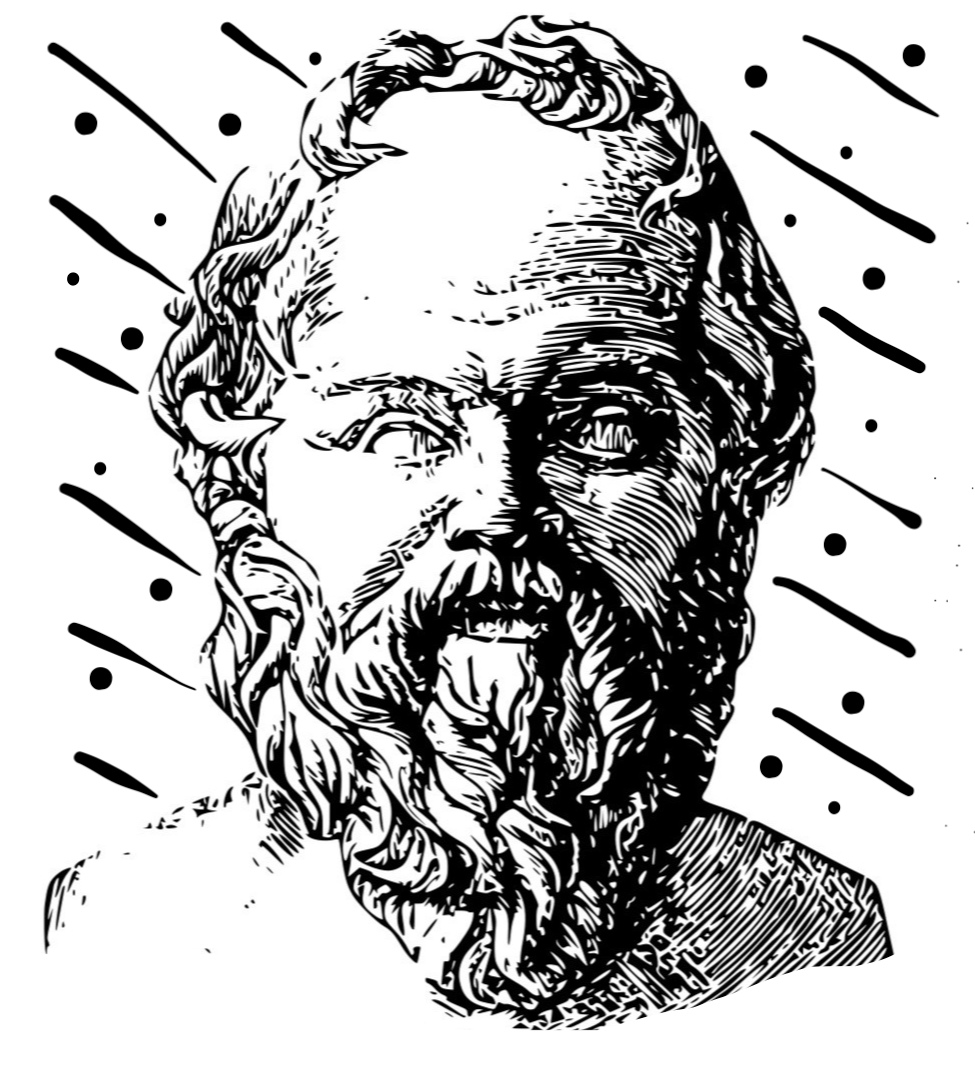Socratic Regression
A Geometric Interpretation of Linear Regression

Socratic Regression is an attempt to develop an intuitive understanding of linear regression using Socratic questioning. The starting point for this project was a geometric proof of the Gauss-Markov theorem which appealed to me as a visual learner. While examining it, I found myself running through lines of questioning in my head which made me realize that Socratic questioning is a more natural way to learn the subject than the traditional ground-up, proof-based approach.

### Linear Regression

Introducing the space and main objects in it

### Ordinary Least Squares

Visualizing how the Gauss-Markov theorem concludes OLS is best